# Magnetic Circuit Analysis

## Magnetic Circuit Analysis:

Magnetic Circuit Analysis – The presence of charges in space or in a medium creates an electric field, similarly the flow of current in a conductor sets up a magnetic field. Electric field is represented by electric flux lines, magnetic flux lines are used to describe the magnetic field. The path of the magnetic flux lines is called the magnetic circuit. Just as a flow of current in the electric circuit requires the presence of an electromotive force, so the production of magnetic flux requires the presence of magneto-motive force (mmf). We now discuss some properties related to magnetic flux.

### (a) Flux density (B):

The magnetic flux lines start and end in such a way that they form closed loops. Weber (Wb) is the unit of magnetic flux (Φ). Flux density (B) is the flux per unit area. Tesla (T) or Wb/m2 is the unit of flux density.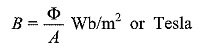where B is a quantity called magnetic flux density in teslas, Φ is the total flux in webers and A is the area perpendicular to the lines in m2.

### (b) Magneto-motive force MMF (ζ):

A measure of the ability of a coil to produce a flux is called the magneto-motive force. It may be considered as a magnetic pressure, just as emf is considered as an electric pressure. A coil with N turns which is carrying a current of I amperes constitutes a magnetic circuit and produces an mmf of NI ampere turns. The source of flux (Φ) in the magnetic circuit is the mmf. The flux produced in the circuit depends on mmf and the length of the circuit.

### (c) Magnetic field strength (H):

The magnetic field strength of a circuit is given by the mmf per unit length.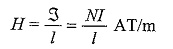The magnetic flux density (B) and its intensity (field strength) in a medium can be related by the following equation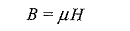where

• μ = μ0μr is the permeability of the medium in Henrys/metre (H/m),
• μ0 = absolute permeability of free space and is equal to 4π x 10-7 H/m and
• μr = relative permeability of the medium.

Relative permeability is a non-dimensional numeric which indicates the degree to which the medium is a better conductor of magnetic flux as compared to free space. The value of μr = 1 for air and non-magnetic materials. It varies from 1,000 to 10,000 for some types of ferro-magnetic materials.

### (d) Reluctance (R):

It is the property of the medium which opposes the passage of magnetic flux. The magnetic reluctance is analogous to resistance in the electric circuit. Its unit is AT/Wb. Air has a much higher reluctance than does iron or steel. For this reason, Magnetic Circuit Analysis used in electrical machines are designed with very small air gaps.

According to definition, reluctance = mmf/flux

The reciprocal of reluctance is known as permeance 1/R = Φ/ζ

Thus reluctance is a measure of the opposition offered by a magnetic circuit to the, setting up of the flux. The reluctance of the magnetic circuit is given by R = 1/μ 1/a.

where I is the length, a is the cross-sectional area of the Magnetic Circuit Analysis and it is the permeability of the medium.

From the above equations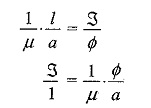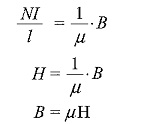Updated: December 28, 2019 — 3:45 pm